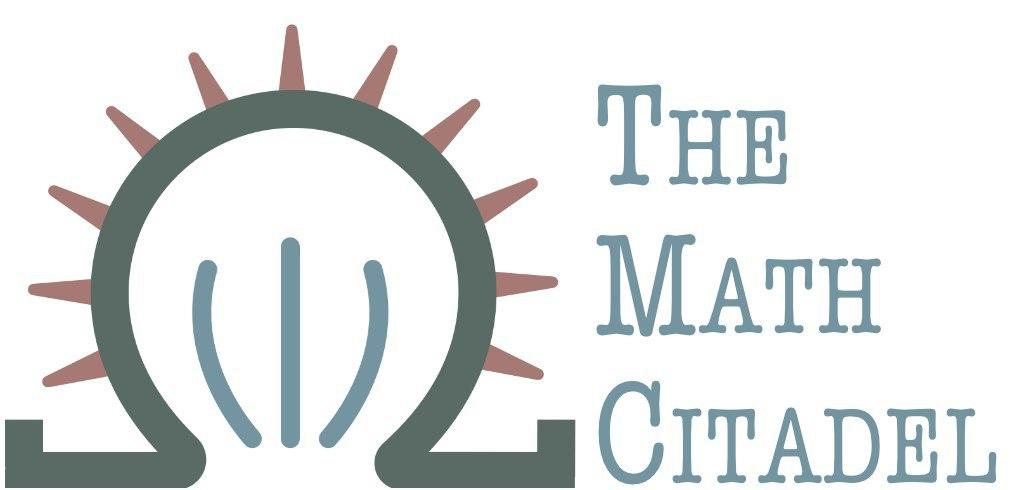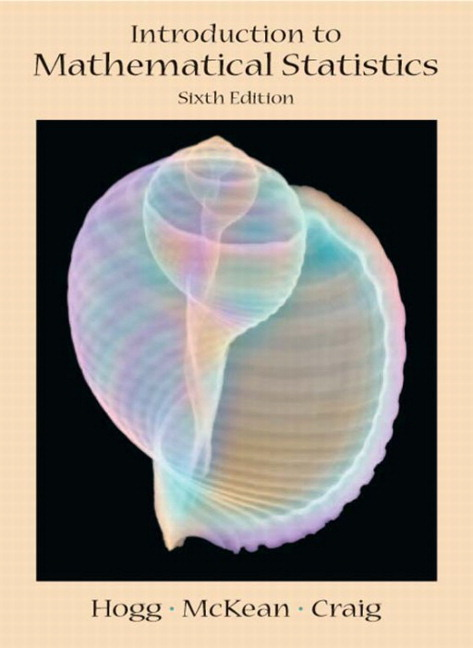## Book Review: Introduction to Mathematical Statistics

### Author: R. Hogg, J. McKean, and A. Craig

#### Reviewed by:## Ranking

All ranking is done on a scale of 1-5, with 5 as the best.
Attribute Rank
Difficulty 3
Proof Quality 4
Self-Study 4
Good for Teaching? 5
Quality of Exercises 5

## Topics Covered

• Basic probability
• Conditional probability, independence
• discrete and continuous random variables, various distributions
• expectation of random variables
• important inequalities (e.g. Chebyshev's inequality)
• Multivarite Distributions
• Bivariate distributions, marginal distributions, conditional distributions
• correlation coefficient
• Special Distributions
• Binomial, Multinomial
• Poisson
• $\Gamma, \chi^{2}, \beta$ distributions
• Normal and multivariate normal
• t and F distributions
• Consistency, Bias, and Limiting Distributions
• Convergence in probability and distribution
• central limit theorem
• Elementary Statistical Inference
• Order statistics
• confidence intervals
• hypothesis testing
• Chi-Square tests
• Monte-Carlo methods
• Bootstrapping
• Maximum Likelihood Methods
• Sufficient Statistics
• Optimal Hypothesis Testing
• Inference on Normal Models
• One-way ANOVA
• noncentral $\chi^{2}$ and F distributions
• Multiple comparisons
• Nonparametric Statistics
• Sign test
• Signed-Rank Wilcoxon test
• Mann-Whitney-Wilcoxon procedure
• general rank tests
• nonparametric measures of association
• Bayesian Statistics
• Linear Models
• Robustness